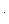## Adding (and Subtracting) Fractions Tips

The “standard” way to add (or subtract) fractions is to put them over a common denominator first. So, the fraction problemis